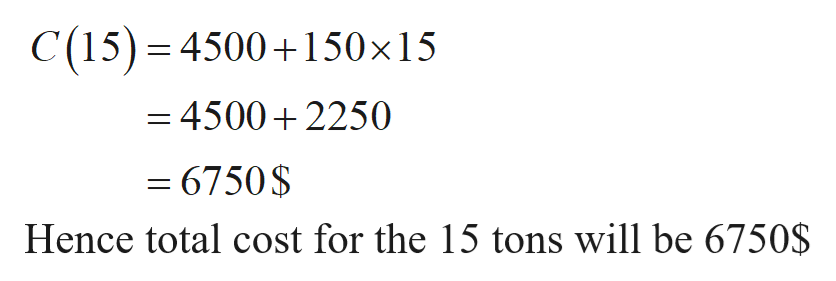# The Sugar Sweet Company is going to transport its sugar to market. It will cost \$4500 to rent trucks plus \$150 for each ton of sugar transported. The total cost, C (in dollars), for transporting n tons is given by the following function.C(n)=4500+150n(a)What is the total cost of transporting 15 tons?(b)If the total cost is \$7800, how many tons is the company transporting?

Question
74 views

The Sugar Sweet Company is going to transport its sugar to market. It will cost \$4500 to rent trucks plus \$150 for each ton of sugar transported. The total cost, C (in dollars), for transporting n tons is given by the following function.

C(n)=4500+150n

 (a) What is the total cost of transporting 15 tons?
 (b) If the total cost is \$7800, how many tons is the company transporting?
check_circle

Step 1

Consider the given information

The total cost function

Step 2

Total cost for 15 tons

Substitute n = 15 in t...help_outlineImage TranscriptioncloseC(15) 4500150x15 - 45002250 - 6750\$ Hence total cost for the 15 tons will be 6750\$ fullscreen

### Want to see the full answer?

See Solution

#### Want to see this answer and more?

Solutions are written by subject experts who are available 24/7. Questions are typically answered within 1 hour.*

See Solution
*Response times may vary by subject and question.
Tagged in

### Other